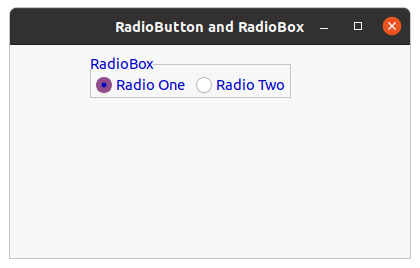# wxPython – Change font colour of RadioBox

• Last Updated : 01 Sep, 2021

In this article we are going to learn how can we change the foreground colour of Radio Box present in the frame. In order to do that we are going to use SetForegroundColour() method. SetForegroundColour() function takes wx.Colour argument which will be used as foreground colour for Radio Box.

Parameters

Code Example:

## Python3

 `import` `wx`  `class` `FrameUI(wx.Frame):` `    ``def` `__init__(``self``, parent, title):``        ``super``(FrameUI, ``self``).__init__(parent, title ``=` `title, size ``=``(``300``, ``200``))` `        ``# function for in-frame components``        ``self``.InitUI()` `    ``def` `InitUI(``self``):``        ``# parent panel for radio box``        ``pnl ``=` `wx.Panel(``self``)` `        ``# list of choices``        ``lblList ``=` `[``'Radio One'``, ``'Radio Two'``]` `        ``# create radio box containing above list``        ``self``.rbox ``=` `wx.RadioBox(pnl, label ``=``'RadioBox'``, pos ``=``(``80``, ``10``), choices ``=` `lblList,``                                          ``majorDimension ``=` `1``, style ``=` `wx.RA_SPECIFY_ROWS)` `        ``# change foreground colour for radio box``        ``self``.rbox.SetForegroundColour((``0``, ``0``, ``255``, ``255``))` `        ``# set frame in centre``        ``self``.Centre()``        ``# set size of frame``        ``self``.SetSize((``400``, ``250``))``        ``# show output frame``        ``self``.Show(``True``)`  `# wx App instance``ex ``=` `wx.App()``# Example instance``FrameUI(``None``, ``'RadioButton and RadioBox'``)``ex.MainLoop()`

Output Window:My Personal Notes arrow_drop_up